## Topic: Multiplication & Division

Free Printable Worksheets Covering Multiplication and Division

## Single Digit Multiplication Worksheet (Set 3)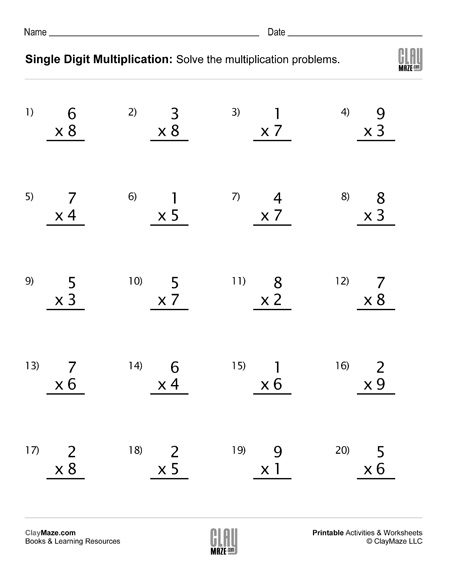Single digit multiplication practice worksheet – 20 problems....

## Single Digit Multiplication Worksheet (Set 4)Single digit multiplication practice worksheet with 20 problems....

## Find the Missing Multipliers Worksheet (Set 1)Free Math Worksheet – Find the Missing Multipliers – 20 problems...

## Find the Missing Multipliers Worksheet (Set 2)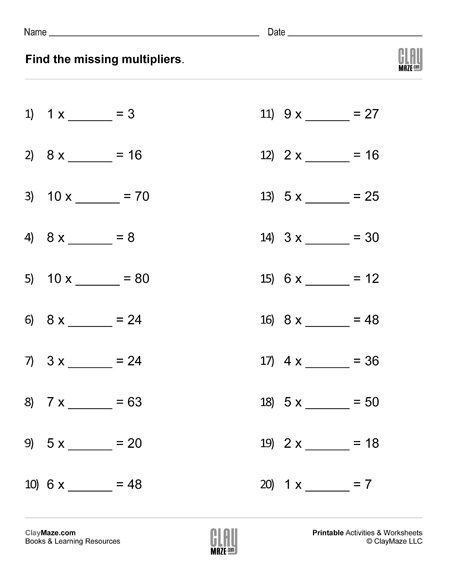Worksheet for multiplication and division practice. – 20 problems...

## Find the Missing Multipliers Worksheet (Set 3)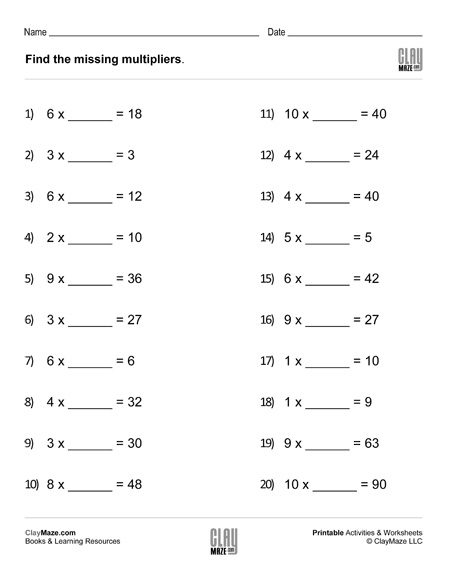Download this worksheet on finding missing multipliers to reinforce  knowledge of multiplication and division facts. – 20 problems...

## Find the Missing Multipliers Worksheet (Set 4)Use this worksheet for practice in multiplication and division facts. – 20 problems...

## Division Worksheet with Single Digit Divisors (Set 1)This is a division worksheet with 5 digit dividends and single digit divisors. They can be solved by either short division or long division. There are no remainders....

## Division Worksheet with Single Digit Divisors (Set 2)This division worksheet can be completed by using either long division or short division. Each problem has a 5-digit dividend and a single digit divisor. – No remainders....

## Division Worksheet with Single Digit Divisors (Set 3)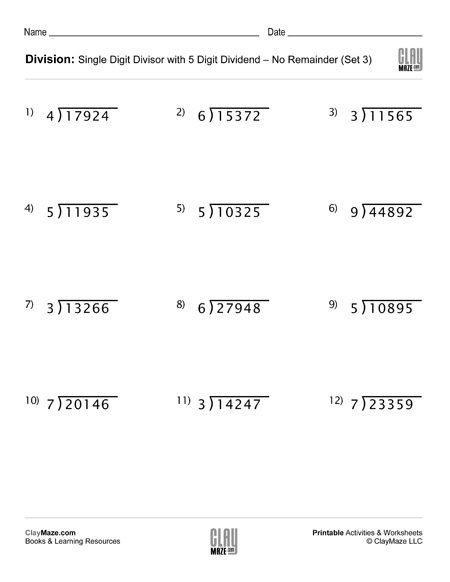This is a worksheet on division practice with a single digit divisor. They can be done using short division or long division. There is plenty of room for either. – No remainders....

## Division Worksheet with Single Digit Divisors (Set 4)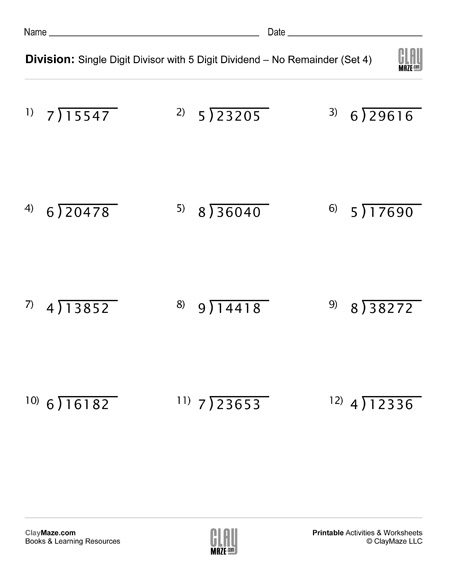Use this worksheet for division practice with single digit divisors. The problems can be solved using either long division or short division. There are no remainders....

## Long Division Worksheet with Double Digit Divisors (Set 1)This worksheet is a set of long division problems with double digit divisors. There are no remainders. – 9 problems...

## Long Division Worksheet with Double Digit Divisors (Set 2)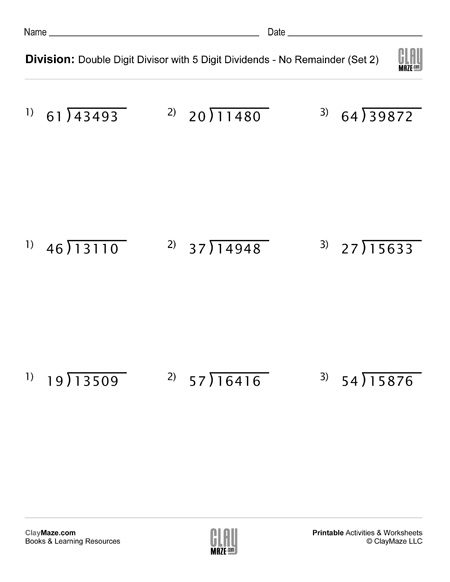This division worksheet is on long division with double digit divisors. There are no remainders. – 9 problems...

## Long Division Worksheet with Double Digit Divisors (Set 3)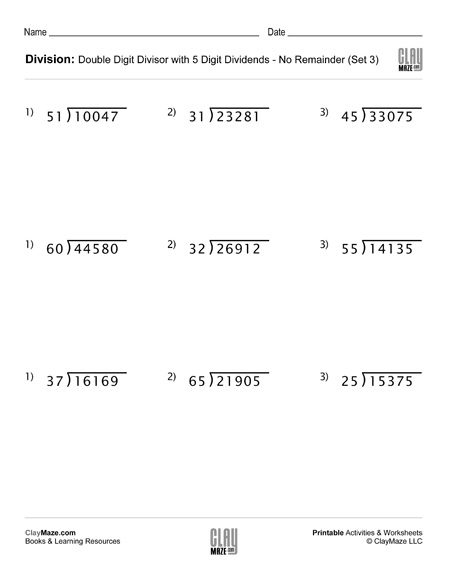Use this worksheet for pracice with long division. The divisors have 2 digits and there are no remainders. – 9 problems...

## Long Division Worksheet with Double Digit Divisors (Set 4)Download this long division practice worksheet. It features double digit divisors and no remainders. – 9 problems...

## Build Your Own Math Problems – Fun Paper Cut OutsThis is a great rainy day activity sheet. This math learning project is a little more interactive in that the child gets to cut out the squares necessary to build the problems and then can either a...